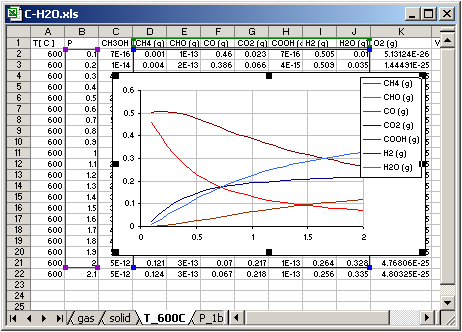AsTher Thermodynamic Database and Applications

AsTher Process Calculator for MS Excel

5.3. Carbon gasification by Water. Create graphics for different variable

5.3.1. Condition Sheet

The green coloured cells are written by user before calculation.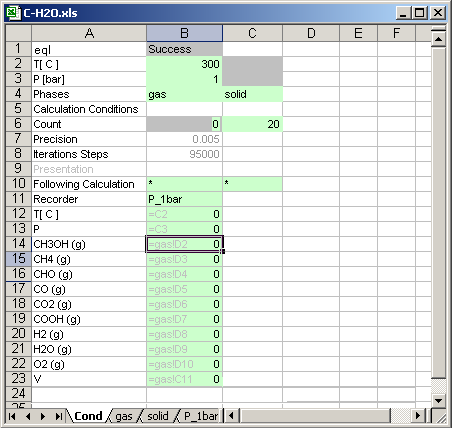Cell [B2]=300+B6*50 means, that after each calculation temperature will be increased in depend of calculation count.
Cell [B3]=1 calculations are carried out for 1 bar.
Cell [B4],[C4] sheet names for the phase is written.

Cell [B6]  0: reset calculations.
Cell [C6]  calculations are carried out 20 times.

Cell [B10] * : means if the calculation count is less than 20, then the next calculations system is defined in the same file.
Cell [C10] * : means if the calculation count is less than 20, then the next calculations system is defined in the same condition sheet.

Cell [B12] P_1bar:Calculations result is written in the sheet named P_1bar, the sheet must exist in the same file (or in the same MS Excel book)

Cells [B13] to [B25]: the cells contain links to another cell in different sheet, which data are recorded in the sheet  P_1bar

5.3.2. Sheet for Phase gas

The green coloured cells are written by user before calculations.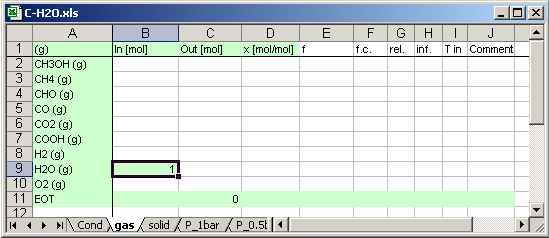Cell [B9] contains input value for H2O (g) 1 [mol].

5.3.3. Sheet for Phase solid

The green coloured cells are written by user before calculations.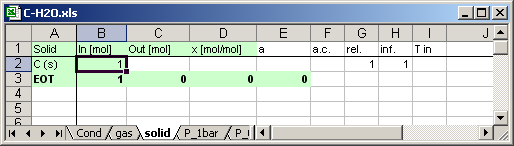Cell [B1] contains input value for C (s) 1 [mol].

5.3.3. Calculations

Execute AsTher Process Calculator for MS Excel (XProCalc.exe).
Select as MS Excel file C-H2O.xls
Select as condition sheet Cond
Press button Calculation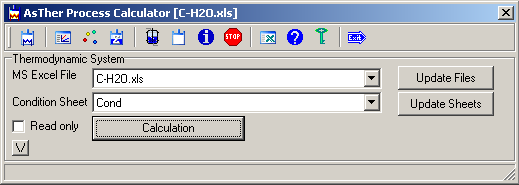5.3.3. Recorded data

5.3.3.1. Calculation results in depend of the temperature

The sheet named P_1bar, contains no any data before calculations.
While calculations, the following data is written by AsTher Process Calculator for MS Excel (XProCalc.exe)in the sheet P_1bar.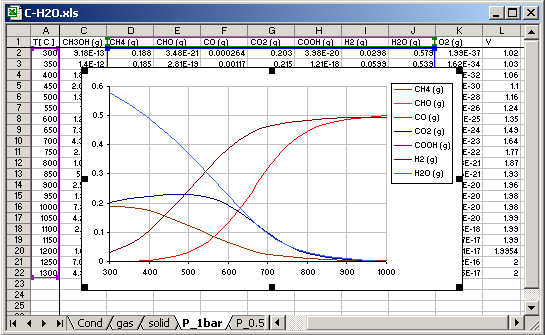Graphic Charts can created by user.

5.3.3.2. Calculation results in depend of the pressure

In the sheet  Cond, the following data are written, before calculations.

Cell [B3]=600 calculations are carried out for 600[C].
Cell [B3]=0.1+B6*0.1 means, that after each calculation pressure will be increased in depend of calculation count.

Cell [B6]  0: reset calculations.
Cell [C6]  calculations are carried out 20 times.

Cell [B13] T_1000C:Calculations result is written in the sheet named T_600C, the sheet must exist in the same file (or in the same MS Excel book)

Cells [B14] to [B25]: the cells contain links to another cell in different sheet, which data are recorded in the sheet  T_600C

The sheet named T_600C, contains no any data before calculations.
While calculations, the following data is written by AsTher Process Calculator for MS Excel (XProCalc.exe) in the sheet T_600C.